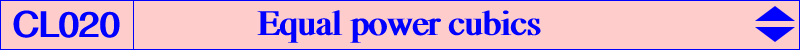The power of M = u:v:w with respect to its pedal circle is given by : P(M) = – 4S^2 uvw / [(u + v + w)(a^2 vw + b^2 wu + c^2 uv)], where S is the area of the reference triangle ABC. The locus of point M such that this power is k (non zero real number) is an isogonal nK with root G and equation : 4S^2 xyz + k(x + y + z)(a^2 yz + b^2 zx + c^2 xy) = 0. All these cubics form a pencil having the same asymptotic directions which are the sidelines of ABC. The three real asymptotes form a triangle homothetic to ABC in the homothety with center K, ratio 1 + k / (S tan w), where w is the Brocard angle. The tangents at A, B, C are the sidelines of the tangential triangle. The most interesting case is K082 obtained with k = – S tan w. Indeed, the ratio of homothety is 0 and the cubic is a nK0+. Each cubic is equivalently the locus of point P whose pedal circle is orthogonal to a fixed (real or not) circle centered at H. When the circle is reduced to the point H, the cubic is K081. For any point M on the cubic K081, we have P(M) = – 4R^2 cosA cosB cosC. Another remarkable cubic of this type is obtained when k = – 1/2 S tan w. It is the locus of M such that the circumanticevian triangle of M and the anticevian triangle of its isogonal conjugate M* are perspective (together with the Grebe cubic K102).